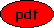A Logic Based Approach to Finding Real Singularities of Implicit Ordinary Differential Equations Co-author(s): Matthias Seiß and Thomas Sturm Reference: Mathematics in Computer Science, 15 (2021) 333-352 Description: This paper is a sequel to  . In  a general framework for the definition and detection of singularities of arbitrary systems of differential equations was developed. Because of the used algebraic algorithms, it was important to consider complex differential equations. In this paper it is shown how at least for ordinary differential equations methods from logic allow to follow the same basic approach also for differential equations over the real numbers. PDF File:(774 kB) Home, Last update: Mon Aug 30 07:58:32 2021